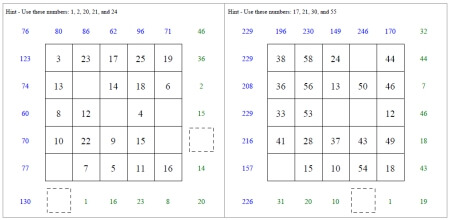Worksheets and No Prep Teaching Resources
Math Worksheets

# Subtraction Squares

Use these creative worksheets with magic subtraction squares to give students a fun, new way to learn their subtraction facts. Students can choose a from variety of different-sized squares, from 3 x 3 up to 5 x 5, as bigger squares give bigger challenges. Some squares even include negative numbers to add to the dynamic thrill of this mathematical game! Students will effortlessly spend time building skills as they have a great time.3 by 3 Magic Subtraction Squares

Numbers 1 to 9 - No Missing Differences
Numbers 1 to 9 - With Missing Differences
Mix of Positive Numbers - With Missing Differences
Mix of Negative Numbers - With Missing Differences

4 by 4 Magic Subtraction Squares

Numbers 1 to 16 - No Missing Differences
Numbers 1 to 16 - With Missing Differences
Mix of Positive Numbers - With Missing Differences
Mix of Negative Numbers - With Missing Differences

5 by 5 Magic Subtraction Squares

Numbers 1 to 25 - No Missing Differences
Numbers 1 to 25 - With Missing Differences
Mix of Positive Numbers - With Missing Differences
Mix of Negative Numbers - With Missing Differences

Super Silly Squares

Multiplication Squares

Mixed Math Square Puzzles

Magic Square Puzzles

Have a suggestion or would like to leave feedback?